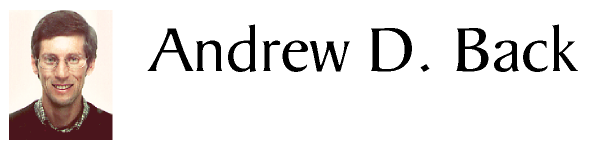Research    Home    Computational Finance    ICA    Hybrid Systems    Recurrent Networks    Support Vector Machines    Input Variable Selection    Classification MethodsPublications    Book Chapters    Journal Papers    Conference Papers    BibTexSoftware    Windale TechnologiesConferences   NIPS*96   NIPS*95   NIPS*94Information   Biography   Contact Me# Input Variable Selection

The problem of input variable selection is well known in the task of modeling real world data. In many real world modeling problems, for example in the context of biomedical, industrial, or environmental systems, a problem can occur when developing multivariate models and the best set of inputs to use are not known.

This is particularly true when using neural networks. In this case, unrequired inputs can significantly increase learning complexity. Input variable selection (IVS) is aimed at determining which input variables are required for a model. The task is to determine a set of inputs which will lead to an optimal model in some sense. Problems which can occur due to poor selection of inputs include the following:
• As the input dimensionality increases, the computational complexity and memory requirements of the model increase.
• Learning is more difficult with unrequired inputs.
• Misconvergence and poor model accuracy may result from additional unrequired inputs.
• Understanding complex models is more difficult than simple models which give comparable results.
The input variable selection method we have developed is based on performing a statistical test between each of the input variable(s) and the desired output from the model. In some situations there may be dependence between input variables which leads to an overestimation of the number of inputs required. One method to overcome this is to use independent component analysis (ICA) as a preprocessing method.

In order to assess the dependence between inputs and the desired system output, we use a method based on higher order cross moments, up to a specified order among the individual terms, and normalized in such a manner as to allow their direct comparison. This statistical measure can be used to establish the independence or otherwise of non-Gaussian signals. These cross moments are defined between the inputs x1,x2,...,xn, individually at time t, and the target output y, with powers up to p=3. Not all cross terms are used, but a selection. The model implements only instantaneous moments, without employing time delays, however it is possible to use lagged regression vectors as inputs to achieve this result. The resulting output is a score vector indicating the dependence of each input on the output. This vector is then classified into to classes using for example, the k-means algorithm to give a binary classification vector.

Because the algorithm uses higher order statistics, it is capable of finding inputs in nongaussian and nonlinear processes.

References
1. A.D. Back and T.P. Trappenberg, "Selecting inputs for modelling using normalized higher order statistics and independent component analysis", IEEE Trans. on Neural Networks, Vol. 12, No. 3, pp. 612-617, May, 2001. Click here to download the paper.

 HomeResearchContact MeFeedbackPublications Copyright © 1989-2008 Andrew Back. All Rights Reserved.